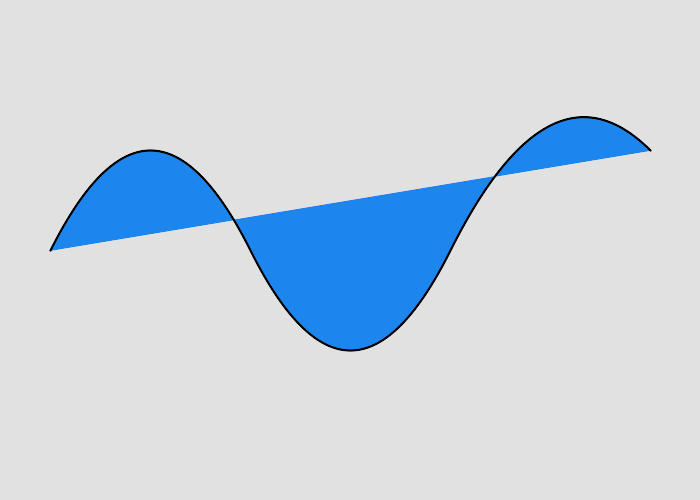Draws a quadratic Bezier curve (using absolute coordinates) from the current point to (x,y). The control point is assumed to be the reflection of the control point on the previous command relative to the current point. (If there is no previous command or if the previous command was not a DrawPathCurveToQuadraticBezierAbsolute, DrawPathCurveToQuadraticBezierRelative, DrawPathCurveToQuadraticBezierSmoothAbsolut or DrawPathCurveToQuadraticBezierSmoothRelative, assume the control point is coincident with the current point.). At the end of the command, the new current point becomes the final (x,y) coordinate pair used in the polybezier.
Please note - quadratic curves are not the same as cubic curves. They cannot be joined smoothly.Background color

Stroke color

Fill color

Draws a quadratic Bezier curve (using absolute coordinates) from the current point to (x,y). The control point is assumed to be the reflection of the control point on the previous command relative to the current point. (If there is no previous command or if the previous command was not a DrawPathCurveToQuadraticBezierAbsolute, DrawPathCurveToQuadraticBezierRelative, DrawPathCurveToQuadraticBezierSmoothAbsolut or DrawPathCurveToQuadraticBezierSmoothRelative, assume the control point is coincident with the current point.). At the end of the command, the new current point becomes the final (x,y) coordinate pair used in the polybezier.
##### Parameters
 x float ending x coordinate y float ending y coordinate